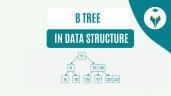How to Use SUBTOTAL in Excel – Shiksha Online

# How to Use SUBTOTAL in Excel – Shiksha Online

clickHere
Updated on Mar 3, 2023 13:26 IST

The article discusses how to use the SUBTOTAL function in Excel.

SUBTOTAL in Excel is used to summarize values and exclude the filtered or hidden rows. The interesting thing is that we can indicate to the SUBTOTAL function the type of operation that we want to apply to the values. If you have a table with sales by region and city, you could calculate the subtotal for each of the regions using the same syntax.

In SUBTOTAL, you can not only calculate the sum, but also use multiple arithmetics, and logical operations like average, maximum, or other available operations. SUBTOTAL function in Excel can be used instead of SUM, COUNT, MAX, etc. to ignore hidden rows, either by filter or manually.

## What is the SUBTOTAL function in Excel?

The SUBTOTAL function in Excel helps us to calculate the subtotal of a list of values. The SUBTOTAL function allows creating a list with the SUBTOTAL command in the Outline group on the Data tab. Once you create the subtotal list, you can easily modify it by just editing the SUBTOTAL Function.

If we take a closer look at the Subtotal dialog box, we can see that we have several options. We will be able to select the field for which we want to make a total, choose one of the eleven available functions, the field to which we want to add the subtotal, replace the current subtotals, add page breaks or include/exclude the summary below the data.

At each change in allows you to select the headers.

Use function allows you to select from functions like SUM, AVERAGE, etc.

This option serves as a kind of wizard for the SUBTOTALS function.

Must Explore – MS Excel Courses

## How to use the SUBTOTAL function in Excel?

The SUBTOTAL function always requires a numeric argument (1 through 11, 101 through 111) as its first argument. This numeric argument is to be applied to subtotals of the values ​​(cell ranges, named ranges) specified as the following arguments.

### Formula for SUBTOTAL in Excel

#### =SUBTOTAL(function_num,ref1,[ref2],…)

To use the SUBTOTAL function, the following parameters must be indicated:

function_num ( Required ): 1 through 11, 101 through 111 indicates the Excel function to use. For example, 1 & 101 = AVERAGE, 9 & 109 = SUM.

ref1 ( Required ): The first range or cell to consider in the operation.

ref2 ( Optional ): The second range or cell to be considered in the operation up to a maximum of 254 ranges.

Note: The numbers 101-111 ignore manually hidden rows, while 1-11 include manually hidden rows.How To Use Slicer In Excel – Shiksha Online
The article discusses the use of Slicer in Excel to sort and filter huge datasetsHow to Create MIS Reports in Excel?
MIS reports are crucial to measure the business performance and contribute towards meeting the overall business goals. In this article, you will learn easy steps to create an MIS report...read moreStandard Deviation In Excel – Shiksha Online
The article discusses standard deviation in Excel.

### Rows Hidden by a Filter

1. For example, we use the SUM function to calculate the total profits monthwise.

2. We will now apply a filter. Here we keep the data just for Jan, Feb, and March. Let’s see how it impacts the total SUM.

We can see that the SUM function only displays the data available in the visible cells.

Related – How To Create A Pivot Table In Excel

3. Now we will apply the SUBTOTAL function, which ignores rows hidden by a filter and calculates the total result.

Where you can see that the AutoComplete feature of Excel allows you to locate the function_num without having to remember it. So in this data set, we need to use the function_num for SUM, which is 109. We will select 109 and then select the cells for calculation.

You can see how the SUBTOTAL function sums up the correct data and ignore the hidden cells.Top MS Excel Interview Questions
Master your Excel interview with confidence using our curated list of top questions. From basic functions to advanced data analysis, boost your Excel proficiency and shine in any data-driven role.Most Popular and Powerful Formulas in Excel
Excel has various functions ready to process your information to get the desired results. Familiarize yourself with each of them. Once you master various functions, you can create advanced MS...read moreHow to Use Solver in Excel – Shiksha Online
The article covers the Solver in Excel. Learn how to use Solver in Excel through examples and facilitate decision-making.

Similarly, you can take out the Average, Count, Standard Deviation, Min & Max, etc. Here I just replaced the function_num to get the Average of the given data.

Press Enter to see the Average of the data set.

### Manually Hidden Rows

1. For example, we can also calculate a range of cells using the SUM function if the rows are hidden using the Hide feature in Excel.

Now we will hide rows manually.

Now we will apply the SUBTOTAL function. The SUBTOTAL function ignores manually hidden rows and calculates the correct result.

The Subtotal Function automatically ignores the manually hidden rows.

Tip – If you are working with very large databases, the best option is to use pivot tables and use slicers to sort and analyze the data.

clickHere

Rashmi is a postgraduate in Biotechnology with a flair for research-oriented work and has an experience of over 13 years in content creation and social media handling. She has a diversified writing portfolio and aim... Read Full Bio

## Trending Management CoursesExcel for Beginners
Great Learning4.6Microsoft Excel - Excel from Beginner to Advanced
UDEMY4.7Excel/VBA for Creative Problem Solving SpecializationExcel/VBA for Creative Problem Solving Specializat...
Coursera5.0## Top Picks & New Arrivals